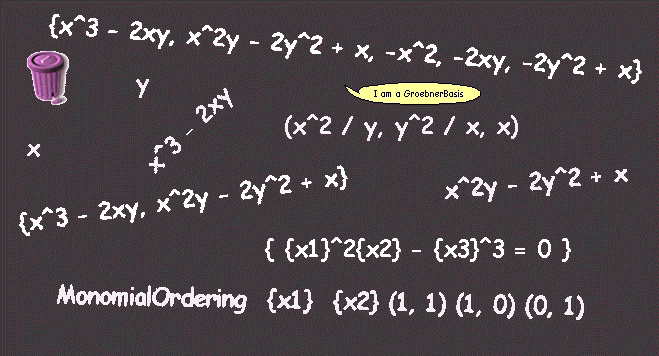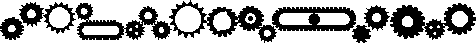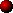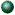Mathematical ObjectsThis is the change set of this work.

The object of this project is to solve the Implicitation Problem, i.e. given an algebraic variety described by a rational parametric equation, find a system of polynomial equations which define it in implicit form.

# Description

We created classes to model multivariate polynomials: MonomialLiteral, Monomial y Polynomial. MonomialLiteral models the literal part of the monomials, i.e., products of indeterminate powers. The Monomials know a coefficient and a literal. Finally, each Polynomial represets a polynomial and stores the collection of not null monomials (Monomials). We put the polynomial division algorithms in different classes: PolynomialDivisor (one variable), PolynomialPseudoDivisor (one or many variables, but w.r.t. a variable), MultiPolynomialDivisor (many variables with fixed monomial ordering). We also have objects which are rational functions; we created the RationalFunction class, this class knows a numerator and a denominator.

# Specification and ImplementationClass

Ideal

You can create ideals from a generating set

Class protocol

instance creation

generator: anIdealGenerator

Also, we can ask an ideal for a generating set accessing generators The ideals allow arithmetic operations

Instance protocol

arithmetic

* anIdeal

+ anIdeal

You can compare ideals trought inclusions

comparing

<= anIdeal

= anIdeal

And we can inquire whether an ideal is null or includes an element

testing

includes: anObject

isNull

Private protocol

accessing-private

generators:This class models polinomial ideals over the rationals using Groebner basis.

Class

IdealWithGroebner

You can create an IdealWithGroebner giving a Groebner basis

Class protocol

instance creation

groebnerBasis: aGroebnerBasis

They can answer a Groebner basis and they can solve the Ideal Membership Problem

Instance protocol

accessing

groebner

testing

includes: aPolynomialThis class models an oredered set of generators.

Class

IdealGenerator

You can add an element to the set, get the n-th element and get the size of the set.

acessing

size

at: anInteger

There are also enumerating messages

enumerating

do: aBlock

collect: aBlock

select: aBlock

You can test the inclusion

testing

includes: anObjectClass

GroebnerBasis

We can create new Groebner bases from a generating set and a monomial ordering or with a default (lexicographic) ordering.

Class protocol

instance creation

from: anIdealGenerator ordering: aMonomialOrdering

from: anIdealGenerator

The Groebner bases can tell the ordering they are using. Furthermore, a basis can be asked for the remainder of the division of a polynomial by the basis, and given a Groebner basis, you can get a minimal one generating the same ideal

Instance protocol

accessing

ordering

remainderOf: aPolynomial

minimal

A Groebner basis can say whether it is minimal or reduced

testing

isMinimal

isReducedIf you want to know the impelmentation, open a browser on the code. Anyway, the following diagram can give you some idea(ls) of the implementation we prefer

Object

MonomialLiteral (contents)

Monomial (literal coefficient)

Polynomial (monomials)

MonomialOrdering (indeterminates weighs)

PolynomialDivisor (dividend divisor quotient remainder done)

PseudoPolynomialDivisor (dividend divisor indeterminate quotient remainder done)

MultiPolynomialDivisor (dividend divisor ordering quotients remainder done)

RationalFunction (numerator denominator)

Ideal (generators)

IdealWithGroebner (groebner)

IdealGenerator (contents)

GroebnerBasis (ordering)

PolynomialEquation (polynomial)

PolynomialSystem (equations)

RationalParametrization (contents)

AlgebraicVariety (system parametrization)

# Example

Here, we are going to see how we can create polynomials. First, we create the indeterminates

x ¬ Polynomial x.

y ¬ Polynomial y.

Then, we see how is the polynomial ordering we are going to use (a general explanation of monomial orderings is in the class comment of MonomialOrdering)

grlex ¬ MonomialOrdering tlex: #(1 2).

MonomialOrdering {x1} {x2} (1, 1) (1, 0) (0, 1)

And then, our polynomials f1 and f2

f1 ¬ x**3 - (2*x*y).

{x1}^3 - 2{x1}{x2}

f2 ¬ x**2*y - (2*y*y) + x.

{x1}^2{x2} - 2{x2}^2 + {x1}

Now, we wish the ideal generating by these polynomials f1 and f2

{{x1}^3 - 2{x1}{x2}, {x1}^2{x2} - 2{x2}^2 + {x1}}

and calculate the Groebner basis

GroebnerBasis from: basis ordering: grlex

{{x1}^3 - 2{x1}{x2}, {x1}^2{x2} - 2{x2}^2 + {x1}, -{x1}^2, -2{x1}{x2}, -2{x2}^2 + {x1}}

Finally, we create a rational parametrization

param ¬ RationalParametrization new.

({x1}^2 / {x2}, {x2}^2 / {x1}, {x1})

and calculate the implicit form

param implicit

{ {x1}^2{x2} - {x3}^3 = 0 }

# ReferencesAdele Goldberg, David Robson, "Smalltalk-80: The Language", Addison-Wesley, 1986.Cox, Little, Oshea, "Ideals, Varieties and Algorithms", Springer-Verlag, 19 .B. Mishra, "Algorithmic Algebra", Springer-Verlag, 19 .Enzo R. Gentile, "Anillo de polinomios", Editorial Docencia, 1980.